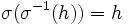# Restriction of automorphism to subgroup invariant under it and its inverse is automorphism

## Statement

Suppose$G$ is a group,$H$ is a subgroup, and$\sigma$ is an Automorphism (?) of$G$ such that both$\sigma$ and$\sigma^{-1}$ leave$H$ invariant. Then,$\sigma$ restricts to an automorphism of$H$.

## Related facts

• Restriction of automorphism to subgroup not implies automorphism: If$\sigma$ is an automorphism of a group$G$ and$H$ is a subgroup of$G$ such that$\sigma(H) \subseteq H$. The restriction of$\sigma$ to$H$ need not be an automorphism of$H$.
• For any group-closed automorphism property (i.e., any property of automorphisms such that for any given group the automorphisms satisfying the property form a group), any subgroup invariant under all automorphisms with the property satisfies the additional condition that the restriction of each such automorphism to the subgroup is an automorphism of the subgroup. A subgroup property that can be expressed this way is termed an auto-invariance property. Examples of this are:
• The property of being a characteristic subgroup is the invariance property with respect to all automorphisms. The restriction of any automorphism of the whole group to a characteristic subgroup is an automorphism of the subgroup.
• The property of being a normal subgroup is the invariance property with respect to all inner automorphisms. The restriction of any inner automorphism of the whole group to a normal subgroup is an automorphism of the subgroup.

## Proof

Given: A group$G$, a subgroup$H$, an automorphism$\sigma$ of$G$ such that$\sigma(H) \subseteq H$ and$\sigma^{-1}(H) \subseteq H$.

To prove:$\sigma(H) = H$ and the restriction of$\sigma$ to$H$ is an automorphism of$H$.

Proof:

1. Since$\sigma$ is an automorphism of$G$, so is$\sigma^{-1}$, and their composite (both ways) is the identity map on$G$. In other words,$\sigma(\sigma^{-1}(g)) = g$ and$\sigma^{-1}(\sigma(g)) = g$ for all$g \in G$.
2. By our assumption, the restrictions$\sigma|_H$ and$\sigma^{-1}|_H$ are both functions from$H$ to itself. Further, we have that$\sigma(\sigma^{-1}(h)) = h$ and$\sigma^{-1}(\sigma(h)) = h$ for all$h \in H$. Thus,$\sigma|_H$ and$\sigma^{-1}|_H$ are two-sided inverses of each other, and are thus both bijections. In particular,$\sigma(H) = H$.
3. Finally, since$\sigma$ is a homomorphism, so is$\sigma|_H$. Thus,$\sigma|_H$ is a bijective homomorphism from$H$ to itself, and is hence an automorphism of$H$.